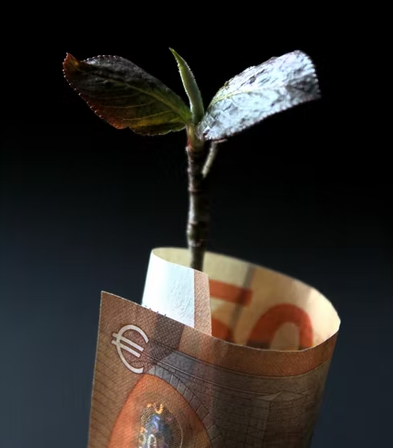## Investment Growth

If €600 is invested today at an annual interest rate of 7% per year, what is its future worth at the end of 35 years?Hint
The question is asking for the future worth, $$F$$ , given the present value/worth, $$P$$ .
Hint 2
Single Payment Compound Amount:
$$F=P(1+i)^n$$$where $$i$$ is the interest rate per interest period, and $$n$$ is the number of compounding periods. The question is asking for the future worth, $$F$$ , given the present value/worth (initial principal balance), $$P$$ . Single Payment Compound Amount: $$F=P(1+i)^n$$$
where $$i$$ is the interest rate per interest period, and $$n$$ is the number of compounding periods.
$$F=€600(1+0.07)^{35}=€600(1.07)^{35}=€6,406$$\$
€6,406